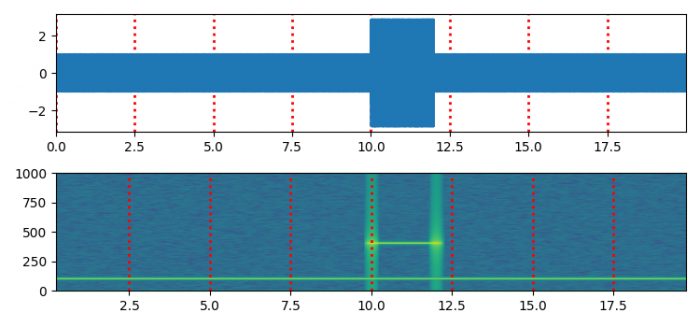# How to place X-axis grid over a spectrogram in Python Matplotlib?

To place X-axis grid over a spectrogram in Python, we can use grid() method and take the following steps −

• Set the figure size and adjust the padding between and around the subplots.
• Create t, s1, s2, nse, x, NEFT and Fs data points using numpy.
• Create a new figure or activate an existing figure using subplots() method with nrows=2.
• Plot t and x data points using plot() method.
• Lay out a grid in current line style.
• Set X-axis margins.
• Plot a spectrogram using specgram() method.
• Lay out a grid in current line style with dotted linestyle and some other properties.
• To display the figure, use show() method.

## Example

import matplotlib.pyplot as plt
import numpy as np

plt.rcParams["figure.figsize"] = [7.50, 3.50]
plt.rcParams["figure.autolayout"] = True

dt = 0.0005
t = np.arange(0.0, 20.0, dt)

s1 = np.sin(2 * np.pi * 100 * t)
s2 = 2 * np.sin(2 * np.pi * 400 * t)
s2[t <= 10] = s2[12 <= t] = 0

nse = 0.01 * np.random.random(size=len(t))
x = s1 + s2 + nse
NFFT = 1024
Fs = int(1.0 / dt)

fig, (ax1, ax2) = plt.subplots(nrows=2)
ax1.plot(t, x)
ax1.grid(axis="x", ls="dotted", lw=2, color="red")
ax1.margins(x=0)

Pxx, freqs, bins, im = ax2.specgram(x, NFFT=NFFT, Fs=Fs, noverlap=900)
ax2.grid(axis="x", ls="dotted", lw=2, color="red")
plt.show()

## Output